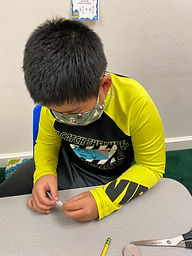Ms. Kylie

Target 1​

Lesson Type:

Continuation

Geometry

:

Symmetry

Identify multiple lines of symmetry in the same figure.

1:

Find multiple lines of symmetry in a figure.

2:

Draw lines of symmetry on given figures.

Define symmetry.

4th

Vocabulary:

Symmetry

Activities:

-Students were given different figures and they folded it and drew the lines of symmetry.

-Students each drew their own figure. Then they switched figures with each other to find the lines of symmetry.Home Exploration

Guiding Questions:Absent Students:

Target 2

:

1:

Explain the arithmetic process used for adding and subtracting fractions with unlike denominators.

2:

Recognize that the first step in adding or subtracting fractions with unlike denominators is to find the least common denominator.

5th

Vocabulary:

Common Denominator, Lease Common Multiple, Mixed Number

Activities:

-Students discussed how to find the common denominator. They practiced finding the least common multiple.

-Students were given several problems that were around the classroom to solve that involved adding mixed fractions with unlike denominators.Home Exploration

Guiding Questions:Target 3

:

Vocabulary:

Activities:Home Exploration